• java中随机函数的使用 方法1 (数据类型)(最小值+Math.random()*(最大值-最小值+1)) 例: (int)(1+Math.random()*(10-1+1)) 从1到10的int型随数方法2 获得随机数
• public class RandomNumber{ ... // 使用java.lang.Math的random方法生成随机数  System.out.println("Math.random(): " + Math.random());  // 使用不带参数的构造方法构造java.util.Random对象


public class RandomNumber{
public static void main(String[] args) {
// 使用java.lang.Math的random方法生成随机数  System.out.println("Math.random(): " + Math.random());
// 使用不带参数的构造方法构造java.util.Random对象  System.out.println("使用不带参数的构造方法构造的Random对象:");  Random rd1 = new Random();  // 产生各种类型的随机数  // 按均匀分布产生整数  System.out.println("int: " + rd1.nextInt());  // 按均匀分布产生长整数  System.out.println("long: " + rd1.nextLong());  // 按均匀分布产生大于等于0，小于1的float数[0, 1)  System.out.println("float: " + rd1.nextFloat());  // 按均匀分布产生[0, 1)范围的double数  System.out.println("double: " + rd1.nextDouble());  // 按正态分布产生随机数  System.out.println("Gaussian: " + rd1.nextGaussian());
// 生成一系列随机数  System.out.print("随机整数序列:");  for (int i = 0; i < 5; i++) {  System.out.print(rd1.nextInt() + " ");  }  System.out.println();
// 指定随机数产生的范围  System.out.print("[0,10)范围内随机整数序列: ");  for (int i = 0; i < 10; i++) {  // Random的nextInt(int n)方法返回一个[0, n)范围内的随机数  System.out.print(rd1.nextInt(10) + " ");  }  System.out.println();  System.out.print("[5,23)范围内随机整数序列: ");  for (int i = 0; i < 10; i++) {  // 因为nextInt(int n)方法的范围是从0开始的，  // 所以需要把区间[5,28)转换成5 + [0, 23)。  System.out.print(5 + rd1.nextInt(23) + " ");  }  System.out.println();  System.out.print("利用nextFloat()生成[0,99)范围内的随机整数序列: ");  for (int i = 0; i < 10; i++) {  System.out.print((int) (rd1.nextDouble()* 100) + "/"); // System.out.println("数学："+(int)(Math.random()*100));  }  System.out.println();  System.out.println();
// 使用带参数的构造方法构造Random对象  // 构造函数的参数是long类型，是生成随机数的种子。  System.out.println("使用带参数的构造方法构造的Random对象:");  Random ran2 = new Random(10);  // 对于种子相同的Random对象，生成的随机数序列是一样的。  System.out.println("使用种子为10的Random对象生成[0,10)内随机整数序列: ");  for (int i = 0; i < 10; i++) {  System.out.print(ran2.nextInt(10) + " ");  }  System.out.println();  Random ran3 = new Random(10);  System.out.println("使用另一个种子为10的Random对象生成[0,10)内随机整数序列: ");  for (int i = 0; i < 10; i++) {  System.out.print(ran3.nextInt(10) + " ");  }  System.out.println();  // ran2和ran3生成的随机数序列是一样的，如果使用两个没带参数构造函数生成的Random对象，  // 则不会出现这种情况，这是因为在没带参数构造函数生成的Random对象的种子缺省是当前系统时间的毫秒数。
// 另外，直接使用Random无法避免生成重复的数字，如果需要生成不重复的随机数序列，需要借助数组和集合类    }  }

展开全文random float string class
• ## Java随机函数

万次阅读 2017-05-12 16:45:09
Java有三种获取随机数的方法 一. java.util.Random包下的Random类，通过new一个Random对象来产生随机数。 二. Math.random()方法，产生的随机数是[0,1)之间的一个double数。 三. System.currentTimeMillis()，该...
Java有三种获取随机数的方法
一. java.util.Random包下的Random类，通过new一个Random对象来产生随机数。
二. Math.random()方法，产生的随机数是[0,1)之间的一个double数。
三. System.currentTimeMillis()，该方法返回从1970年1月1号0点0分0秒到现在的毫秒数，返回值为long。

Random类
Random类有两种构造方法：带种子和不带种子。
不带种子:
每次运行结果不一样。默认种子为System.currentTimeMillis()，即系统时间作为seed。因为时间一直在改变所以种子也在变。
Random() rdm = new Random();
//Random() rdm = new Random(System.currentTimeMillis());

带种子:
每次运行结果都一样。相同种子的运行结果一样。
Random rdm = new Random(100);

Random类中的8种方法

rdm.nextInt()  //返回int取值范围内的伪随机数，[2147483648 , 2147483647]
rdm.nextInt(n)  //返回0~n的伪随机数，[0 , n)
rdm.nextLong()//返回long取值范围内的伪随机数，[-(2^63-1) , 2^63]
rdm.nextDouble()  //返回double类型的伪随机数，[0.0 , 1.0)
rdm.nextFloat()  //返回float类型的伪随机数，[0.0 , 1.0)
rdm.nextBytes(bytes)//用随机数填充指定字节数组的元素
rdm.nextBoolean()//返回true或false
rdm.nextGaussian()//返回高斯/正态分布

接下来对以上方法进行测试

import java.util.Random;

public class TestRandom2 {

public static void main(String[] args) {

Random rdm1 = new Random();//不带种子
Random rdm2 = new Random(100);//带种子
Random rdm3 = new Random(100);//与rdm2带一样的种子

byte[] data = new byte;
//用随机数填充data数组
rdm1.nextBytes(data);

for(int i=0;i<3;i++){
System.out.println("不带种子的nextInt(): "+rdm1.nextInt());
}
System.out.println();
for(int i=0;i<3;i++){
System.out.println("不带种子的nextInt(10): "+rdm1.nextInt(10)+",");
}
System.out.println();
for(int i=0;i<3;i++){
System.out.println("rdm2带种子数100: "+rdm2.nextInt(10));
}
System.out.println();
for(int i=0;i<3;i++){
System.out.println("rdm3带种子数100: "+rdm3.nextInt(10));
}
System.out.println();

System.out.println("nextLong(): "+rdm1.nextLong());
System.out.println("nextDouble(): "+rdm1.nextDouble());
System.out.println("nextFloat(): "+rdm1.nextFloat());
System.out.println("nextGaussian(): "+rdm1.nextGaussian());
System.out.println("nextBoolean(): "+rdm1.nextBoolean());
System.out.print("nextBytes(bytes): ");
for(int i=0;i<3;i++){
System.out.print(data[i]+" ");
}
}
}

第一次运行结果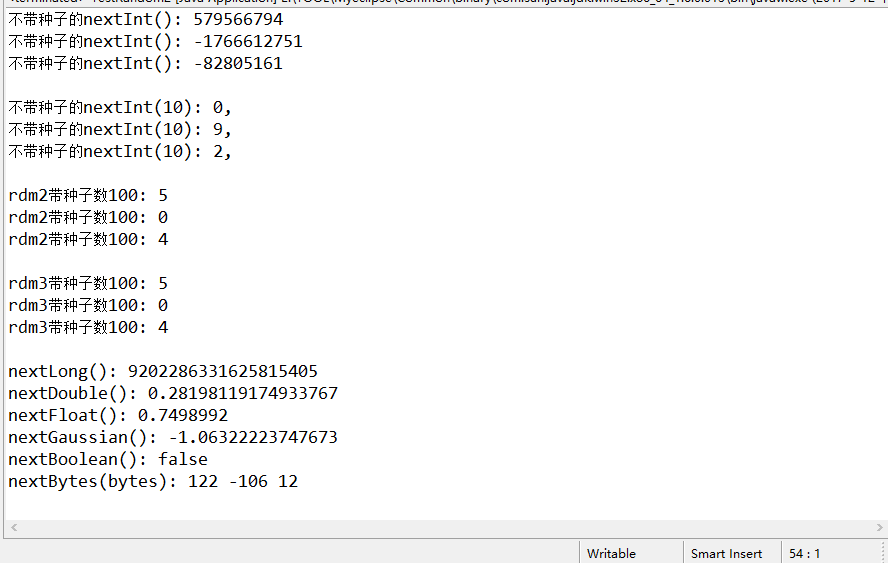第二次运行结果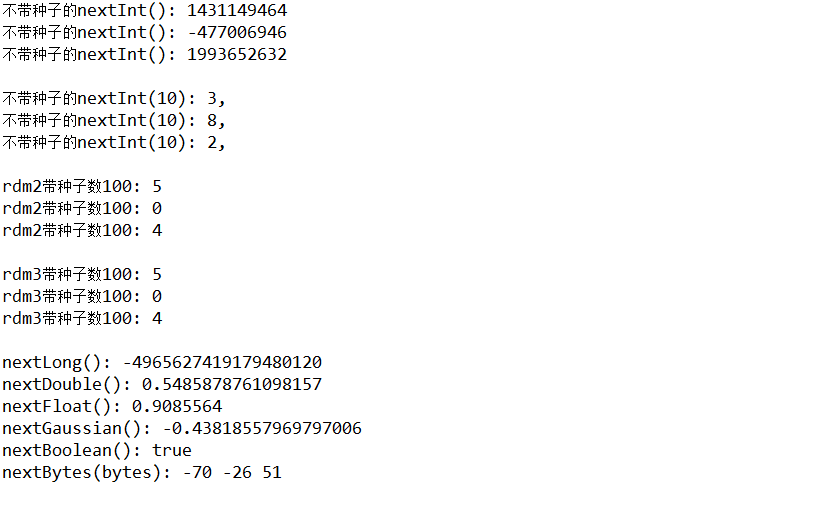可以看出带种子的构造方法，两次运行结果是一样的。种子数相同运行结果也是一样的。
不带种子的两次运行结果则不同。

Math.random()

产生[0,1)之间的double类型随机数

Math.random()*n>>>[0,n)之间的随机数

(int)(Math.random()*n)>>>[0,n)之间的随机整数  //int后面的()不能缺，否则会先强制转换Math.random()

Math.round(Math.random()*(MAX-MIN)+MIN)>>>[MIN,MAX]//Math.round()可以暂时理解为四舍五入函数

进行测试

double temp1;
int temp2;
long temp3;

System.out.print("[0,1): ");
for(int i=0;i<3;i++){
temp1=Math.random();
System.out.print(temp1+" ");
}
System.out.println();
System.out.print("[0,9]: ");
for(int i=0;i<3;i++){
temp2=(int) (Math.random()*10);
System.out.print(temp2+" ");
}
System.out.println();
System.out.print("[0,10]: ");
for(int i=0;i<3;i++){
//Math.round 四舍五入函数(负数的话小数等于5时不进位，-1.5=-1)
temp3=Math.round(Math.random()*9+1);
System.out.print(temp3+" ");
}

运行结果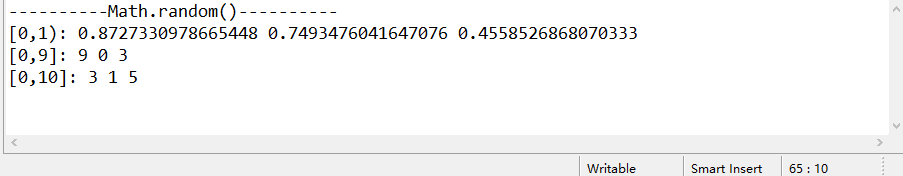System.currentTimeMillis()

返回当前的系统时间，返回值为long

temp=System.currentTimeMillis()%100;  //对系统时间取模，返回[0,99]之间的随机整数

此方法有个很大的缺陷，当两次取值的时间间隔非常小时，取出的值是相同的。

因此我们需要在循环中加入sleep。

进行测试

long r1,r2;

System.out.print("返回当前毫秒数： ");
for(int i=0;i<3;i++){
r1 = System.currentTimeMillis();
System.out.print(r1+" ");
}
System.out.println();

System.out.print("加入sleep(): ");
for(int i=0;i<3;i++){
r1=System.currentTimeMillis();
System.out.print(r1+" ");
try {
} catch (InterruptedException e) {
e.printStackTrace();
}
}
System.out.println();

//对系统时间进行模运算
System.out.print("一百以内随机数: ");
for(int i=0;i<3;i++){
r2=System.currentTimeMillis()%100;
System.out.print(r2+" ");
}
System.out.println();

//sleep后的模运算
System.out.print("加入sleep(): ");
for(int i=0;i<3;i++){
r2=System.currentTimeMillis()%100;
System.out.print(r2+" ");
try {
} catch (InterruptedException e) {
e.printStackTrace();
}
}

运行结果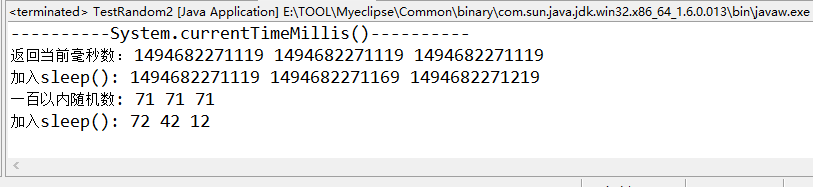展开全文random 函数
• ## java随机函数

千次阅读 2019-03-09 23:18:34
看了下以前笔记，特此记录下 Java math类random函数取值在0.0-0..99 Java.util.Random类 生成一个随机数
看了下以前笔记，特此记录下
Java math类random函数取值在0.0-0..99
Java.util.Random类 生成一个随机数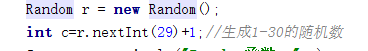展开全文• 今天在做Java练习的时候注意到了Java里面的一个随机函数——Random，刚开始只是知道这个函数具有随机取值的作用，于是上网搜索了资料一番，做了一下一些关于Random函数的总结： Java中存在着两种Random函数： ...

文章原文地址：http://blog.sina.com.cn/s/blog_93dc666c0101h3gd.html  今天在做Java练习的时候注意到了Java里面的一个随机函数——Random，刚开始只是知道这个函数具有随机取值的作用，于是上网搜索了资料一番，做了一下一些关于Random函数的总结：

Java中存在着两种Random函数：

一、java.lang.Math.Random;

调用这个Math.Random()函数能够返回带正号的double值，该值大于等于0.0且小于1.0，即取值范围是[0.0,1.0)的左闭右开区间，返回值是一个伪随机选择的数，在该范围内（近似）均匀分布。

例如下面的实验代码编译通过后运行结果如下图观察会发现代码的用一个循环10次循环输出num的取值，均随机分布在[0,3)之间！在使用Math.Random()的时候需要注意的地方时该函数是返回double类型的值，所以在要赋值给其他类型的变量的时候注意需要进行塑形转换。

二、java.util.Random;

在Java的API帮助文档中，总结了一下对这个Random()函数功能的描述：

1、java.util.Random类中实现的随机算法是伪随机，也就是有规则的随机，所谓有规则的就是在给定种(seed)的区间内随机生成数字；

2、相同种子数的Random对象，相同次数生成的随机数字是完全相同的；

3、Random类中各方法生成的随机数字都是均匀分布的，也就是说区间内部的数字生成的几率均等；

下面Random()的两种构造方法

1.Random()：
创建一个新的随机数生成器。

2.Random(longseed)：
使用单个 long种子创建一个新的随机数生成器。

我们可以在构造Random对象的时候指定种子（这里指定种子有何作用，请接着往下看），如：

Random r1 = new Random(20);

或者默认当前系统时间对应的相对时间有关的数字作为种子数:

Random r1 = new Random();

需要说明的是：你在创建一个Random对象的时候可以给定任意一个合法的种子数，种子数只是随机算法的起源数字，和生成的随机数的区间没有任何关系。如下面的Java代码：

Random rand =new Random(25);

int i;

i=rand.nextInt(100);

初始化时25并没有起直接作用（注意：不是没有起作用）,rand.nextInt(100);中的100是随机数的上限,产生的随机数为0-100的整数,不包括100。

下面是Java.util.Random()方法摘要

1.protectedint next(intbits)：生成下一个伪随机数。

2.boolean nextBoolean()：返回下一个伪随机数，它是取自此随机数生成器序列的均匀分布的boolean值。

3.void nextBytes(byte[]bytes)：生成随机字节并将其置于用户提供的 byte 数组中。

4.double nextDouble()：返回下一个伪随机数，它是取自此随机数生成器序列的、在0.0和1.0之间均匀分布的double值。

5.float nextFloat()：返回下一个伪随机数，它是取自此随机数生成器序列的、在0.0和1.0之间均匀分布float值。

6.double nextGaussian()：返回下一个伪随机数，它是取自此随机数生成器序列的、呈高斯（“正态”）分布的double值，其平均值是0.0标准差是1.0。

7.int nextInt()：返回下一个伪随机数，它是此随机数生成器的序列中均匀分布的int 值。

8.int nextInt(intn)：返回一个伪随机数，它是取自此随机数生成器序列的、在（包括和指定值（不包括）之间均匀分布的int值。

9.long nextLong()：返回下一个伪随机数，它是取自此随机数生成器序列的均匀分布的long 值。

10.void setSeed(longseed)：使用单个 long 种子设置此随机数生成器的种子。

方法摘要也就这些，下面给几个例子：

1.生成[0,1.0)区间的小数：double d1 =r.nextDouble();

2.生成[0,5.0)区间的小数：double d2 = r.nextDouble()* 5;

3.生成[1,2.5)区间的小数：double d3 = r.nextDouble()* 1.5 + 1;

4.生成-231到231-1之间的整数：int n =r.nextInt();

5.生成[0,10)区间的整数：

int n2 = r.nextInt(10);//方法一

n2 = Math.abs(r.nextInt() %10);//方法二

前面曾讲到过构造Random对象的时候指定种子的问题，到底指定种子有什么作用呢，这里直接用代码例子来做说明：在定义的时候分别指定了相同的种子之后，在分别用r1和r2去[0,30)的随机数，结果编译执行后悔发现结果都是呈现AABB型的，说明r1和r2取的随机数是一模一样的（下图为实验截图）。如果我改动代码，改成下面这样：再编译输出后，就再也不会得到AABB型的结果，根据代码的区别，就可以知道指定种子数，和不指定种子数的区别在于哪里了。最后再来简单对比一下这两个随机函数到底的特点：

1.java.Math.Random()实际是在内部调用java.util.Random()的,它有一个致命的弱点，它和系统时间有关，也就是说相隔时间很短的两个random比如:

double a = Math.random()；

double b = Math.random();

即有可能会得到两个一模一样的double。

2.java.util.Random()在调用的时候可以实现和java.Math.Random()一样的功能，而且他具有很多的调用方法，相对来说比较灵活。所以从总体来看，使用java.util.Random()会相对来说比较灵活一些。


展开全文• ## java随机函数

千次阅读 2014-12-06 00:30:21
Java中存在着两种Random函数： 一、java.lang.Math.Random; 调用这个Math.Random()函数能够返回带正号的double值，该值大于等于0.0且小于1.0，即取值范围是[0.0,1.0)，返回值是一个伪随机选择的数，在该范围内...
• ## 随机函数JAVA

万次阅读 2018-07-20 00:12:23
接下来开始我们随机函数的代码块 package first; import java.util.StringTokenizer; public class First {  public static void main(String[]args) {    /*随机验证码的编写*/    String r...
• ## java随机函数的使用

千次阅读 2019-03-18 23:46:04
int nums = (int)num1 + (int)(Math.random()*(num2-num... // 这一步只是生成num1 到num1+100的一个随机偶数 if (nums % 2 == 0) { arraynum[i] = nums; } else { arraynum[i] = nums + 1; } }
• import java.util.Random; public class RandomNumber{ public static void main(String[] args) { // 使用java.lang.Math的random方法生成随机数 System.out.println("Math.random(): " + Math....random float import string
• 利用随机函数产生16个1~10的随机整数给一个4*4的二维数组。 （1）二维数组按行列输出； （2）求最外一圈元素之和； （3）求主对角线最大元素的值及其位置。 二、实验代码 问题解释详见代码注释。 package fighting...
• public void suiji() { int m,n,k=0,k1,k2,k3; for(m=1;m;m++) { k1=(int)(Math.random()*25+1); for(n=1;n;n++) { k2=(int)(Math.random()*6+1); k3=(int)(Math.random()*5+1); while(d[k2][k3])!... }
• 此函数适用于获取指定范围的随机函数，包括整数和小数(比如获取小数点后两位的小数，只要最后结果除以100，剩下的方法依此类推) Random random = new Random(); int s = random.nextInt(max)%(max-min+1) + minrandom
• 用数组和随机函数编程： 生成一个4*4的二维数组 编写一个方法appear，模拟在二维数组的任意位置出现地鼠，并打印地鼠出现位置(用o表示)。 地鼠生成后，地鼠出现位置标记为X，并无法再次产生地鼠 二维数组被X占满后...
• 利用随机函数产生 20 个 10~90 之间的不重复整数。 将这些数拼接在一个字符串，用逗号隔开。 每产生一个新数，要保证在该字符串不存在。 最后将串的整数分离存放到一个数组，将数组的内容按由小到大输出。 ...字符串
• ## Java随机 Java真随机

千次阅读 2016-12-30 14:17:56
最近发现java自带的随机函数是可以遇见的，自己就根据随机的理解利用cpu和时间的随机种子写了一个随机类，希望对你有所启发！！！ 不说这么多了直接上源码：public class Random { public static void main(String...线程
• Java中的Random（）函数   Java中存在着两种Random函数： 一、java.lang.Math.Random; 调用这个Math.Random()函数能够返回带正号的double值，该值大于等于0.0且小于1.0，即取值范围是[0.0,1.0)的左闭右开区间...
• Java中Random()对象是生成随机数的对象。Random()有两种构造方法： Random()：创建一个新的随机数生成器，这种方式采用默认的种子。 Random(long seed)：使用seed为种子创建一个新的随机数生成器。 种子的作用...生成区间内的随机数
• 1、java.util.Random类实现的随机算法是伪随机，也就是有规则的随机，所谓有规则的就是在给定种(seed)的区间内随机生成数字； 2、相同种子数的Random对象，相同次数生成的随机数字是完全相同的； 3、Random类各...random 对象
• 主要介绍了java实现随机生成UUID的函数代码，有需要的小伙伴可以参考下。
• 1、生成的字符串每个位置都有可能是str的一个字母或数字，需要导入的包是import java.util.Random; //length用户要求产生字符串的长度 public static String getRandomString(int length){ String str=...
• ## Java中的Random()函数

千次阅读 2018-01-23 20:50:19
Java中的Random()函数 Java中存在着两种Random函数： 一、java.lang.Math.Random: 调用这个Math.Random()函数能够返回带正号的double值，该值大于等于0.0且小于1.0，即取值范围是[0.0,1.0)的左闭右...
•  java.lang.Math类包含基本的数字操作，如指数、对数、平方根和三角函数。  java.math是一个包，提供用于执行任意精度整数(BigInteger)算法和任意精度小数(BigDecimal)算法的类。    java.lang.Math类...数学计算 math
• ## java中的数学函数

千次阅读 2008-12-19 17:44:00
java中的数学函数调用Java中的数学函数需要使用 import java.lang.Math.*;ceil(double)返回不小于该参数的最小整数 (最接近负无穷)的 double 型值。floor(double)返回不大于该参数的最大整数 (最接近正无穷)的 ...math random import
• Java常用数学函数总结及求数组的平均数、位数和众数
•  今天在做Java练习的时候注意到了Java里面的一个随机函数——Random，刚开始只是知道这个函数具有随机取值的作用，于是上网搜索了资料一番，做了一下一些关于Random函数的总结： Java中存在着两种Random函数...
• 扫描二维码，关注公众号 ...Java中产生随机数 1 . 调用java.lang下面Math类的random()方法产生随机数 public class MyRandom { public static void main(String[] args) { int radom = (i...random 存储 boolean
• java 存在两个随机函数 1. 来自 java.lang.Math.random() 2.来自java.lang.Math.random() 1.先说java.lang.Math.random(): 调用Math.Random()函数能够返回带正号的double值，该值大于等于0.0小于1.0，返回一...random
• 题目： 本程序通过随机产生一个三角形，然后从控制台输入一个点P，结果判断点P是否在三角形内。 思路：是根据点P计算三角形的面积，来判断点是否在三角形内部。 package sunquan; import java.math.*; import java......

# java中随机函数java 订阅